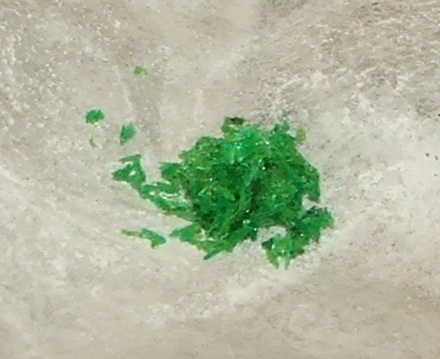Q1+2: When calcium carbonate is added to hydrochloric acid a gas is given off and calcium chloride is formed.

1. What is the test for the gas produced?

• A.   bleaches damp litmus paper
• B.   pops with a lit splint
• C.   relights a glowing splint
• D.   turns limewater milky
2. What is the formula of calcium chloride?
• A.   CaCl
• B.   Ca2Cl
• C.   CaCl2
• D.   Ca2Cl2
3. Dilute hydrochloric acid reacts with sodium hydroxide solution.

What type of reaction is this and which ionic equation correctly represents this reaction?

 Reaction Type Ionic Equation A displacement H+(aq) + OH-(aq) → H2O B neutralisation H+(aq) + OH-(aq) → H2O C displacement Cl-(aq) + Na+(aq) → NaCl(aq) D neutralisation Cl-(aq) + Na+(aq) → NaCl(aq)
4. Which statement about ion concentrations in alkalis is true?

An alkali contains..

• A.   a greater concentration of hydroxide ions than pure water
• B.   a greater concentration of hydrogen ions than pure water
• C.   equal concentrations of hydrogen and hydroxide ions
• D.   the same concentration of hydroxide ions as pure water
5. A small amount of ethanoic acid is dissolved in a large volume of water. What is the best description of this solution?
• A.   A dilute solution of a strong acid
• B.   A weak solution of a concentrated acid
• C.   A dilute solution of a weak acid
• D.   A weak solution of a dilute acid
6. A solution has a pH of 5. What is the pH of the solution if the hydrogen ion concentration increases by a factor of 100?
• A.   3
• B.   4
• C.   6
• D.   7

Q7-8:
Some soluble salts such as copper(II)chloride are prepared by adding excess insoluble solid to acid.7. Why do you add excess of the insoluble solid?
• A.   To ensure that a reaction takes place
• B.   To speed up the reaction
• C.   To allow the solid to be separated from the solution
• D.   To ensure that all the acid is used up
8. Which technique is used to separate the excess insoluble solid from the reaction mixture?
• A.   crystallization
• B.   filtration
• C.   precipitation
• D.   titration

9. Which of the following would produce a precipitate when they are mixed together?

 I. Sodium chloride and silver nitrate solutions II. Copper(II)sulfate and sodium hydroxide solutions III. Potassium carbonate and magnesium nitrate solutions

• A.   I and II only
• B.   I and III only
• C.   II and III only
• D.   I, II and III

10. There are three different methods for preparing pure, dry samples of a salt:

 1. Adding an excess insoluble solid to acid 2. Adding alkali to acid 3. Precipitation

Which method is most suitable to prepare each of the salts in the table below?

 Lead(II)sulfate Copper(II)sulfate ammonium sulfate A 3 2 1 B 1 2 3 C 2 1 3 D 3 1 2